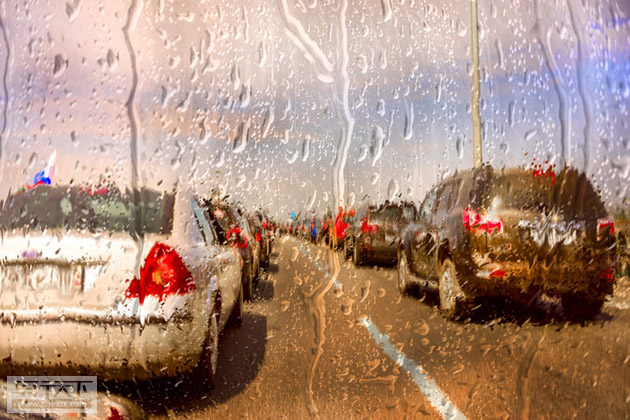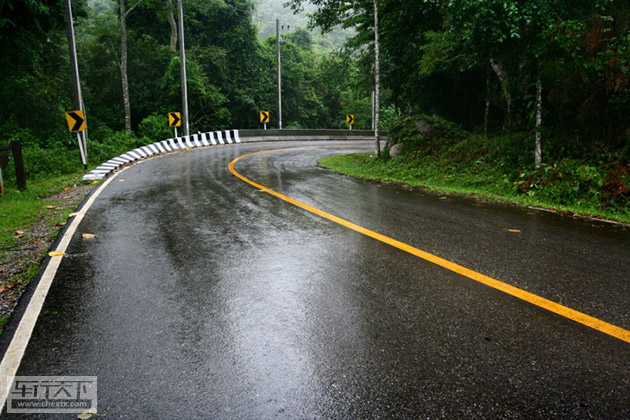## 瓜子二手车教你做好春雨前的七个部位保养

2017年03月17日 09:28 来源：www.chextx.com 超过：次关注

虽说春雨贵如油，但下雨天却着实令很多车主感到头疼，行驶时视线受到影响不说，还容易造成车内潮湿、车外生锈等各种各样的问题。在雨季完全来临之前，瓜子二手车建议车主们多注意以下几个部位，提前把这些部分的保养做起来，以便顺利度过雨季。1、发动机和排气管

在开车行驶的途中遇到路面有积水的情况时，可千万别大大咧咧地冲过去，一定要先停车观察一下水的深度，如果遇见深水，又没办法绕道，可以将橡胶管接到排气管上，然后将管口向上，这样能在一定程度上保证发动机和排气管的安全。

即便积水不深，也应该缓慢地行驶过去，避免激起太多水花进入发动机和排气管，要是因此导致爱车出现问题可就得不偿失了。

2、车漆

雨水中的酸性成分对汽车的漆面具有极强的腐蚀作用，时间长了会对漆面造成损害。因此在雨水较多的季节来临之前，最好能给爱车进行一次漆面修容，最简单的是做打蜡处理，更长久、有效的是进行封釉或镀膜。打蜡可以自己做，也可以交给4S店，但是封釉和镀膜非常专业和复杂，就必须交由专业机构解决了。

3、底盘

底盘是和地面最贴近的部位，因此深受路况影响，既要避免磕碰，还要避免生锈。从全车来看，一般底盘是最容易锈蚀斑斑的，轮壳处甚至还可能松动穿孔。因此，一定要注意汽车底盘的清洁和防锈，做一次底盘防锈处理，防锈效果一般可保持3个月时间。

4、点火系统

很多车主会遇到雨天打不着火的问题，即使勉强启动起来，也是有气无力的。引起这种问题最主要的原因可能是出自点火系统因潮湿而产生漏电情况，此外，还有可能是因为雨天天气变凉，而封在分电盘内的热空气就有可能凝结成水滴而造成分电盘内部的小水灾，因而丧失了正常的点火功能。

如果只是因为雨水将点火点弄得有点湿而点不着火，可以试试用干纸巾或者干布把打火线擦干，再用一些干燥剂喷在火花塞、点火线圈等地方，等几分钟使其干燥后，车子一般就可以启动了！5、雨刮器

雨天的路面及视线都不利于驾驶，如果雨刮器不能很好刷掉雨水，会给行车安全带来很大危险，雨水的增多必然让雨刮器承担更多的工作，因此对它的检查保养要更加细心到位。

平常要对雨刷器做详细的检查，如刮水效果是否良好，是否有震动或者异响，雨刷器上的橡胶片是否已发生老化？一旦发现上述情况，就需要及时更换了，以免因视野带来安全问题。

6、音响系统

雨季来临湿度变大，CD表面会开始出现雾气，造成读盘困难，因此最好每隔一段时间擦干一次。在安装CD驱动器时，为了避免潮湿，不要安装在底板和座位下面，最好安装在车身的高处，但是要避免安装在后风挡玻璃处，以免温度过高加速电子元件和激光头的老化。

7、车内

在车内防潮方面，最“便宜”的办法就是带爱车晒太阳。找个好天，将爱车停在太阳下，打开四门车窗，让室内空气对流一番，被晒热的车身很快就会排除内部淤积的水汽了。如果难得晴天，开冷气是最好的方法，即使气温较低，只要设定室内温度，同时开启冷气并不会减少暖气的效果，反而可以起到除湿的效果。

#### 相关文章

﻿
• 快速找车
• 选择品牌
• 选择品牌
• A  奥迪
• A  阿斯顿·马丁
• A  阿尔法·罗密欧
• B  宝沃
• B  布加迪
• B  巴博斯
• B  保时捷
• B  宾利
• B  奔驰
• B  宝马
• B  本田
• B  别克
• B  标致
• B  比亚迪
• B  宝骏
• B  北汽制造
• B  北汽新能源
• B  北汽幻速
• B  北汽威旺
• B  北京汽车
• B  奔腾
• B  北汽绅宝
• B  北汽昌河
• C  长安欧尚
• C  长安
• C  长安凯程
• C  长城
• D  大众
• D  道奇
• D  DS
• D  东南
• D  东风风神
• D  东风风行
• D  东风小康
• D  东风风度
• D  东风
• F  福特
• F  丰田
• F  菲亚特
• F  法拉利
• F  福田
• F  福迪
• F  福汽启腾
• G  观致
• G  广汽传祺
• G  广汽吉奥
• G  GMC
• H  红旗
• H  汉腾汽车
• H  哈弗
• H  哈飞
• H  海格
• H  海马
• H  华颂
• H  黄海
• H  华泰
• H  恒天
• J  捷途
• J  几何汽车
• J  捷达
• J  吉利汽车
• J  捷豹
• J  Jeep
• J  江淮
• J  江铃
• J  金杯
• J  九龙
• J  金旅
• K  凯翼
• K  凯迪拉克
• K  克莱斯勒
• K  科尼塞克
• K  卡威
• K  开瑞
• L  路虎
• L  林肯
• L  劳斯莱斯
• L  兰博基尼
• L  雷克萨斯
• L  铃木
• L  领克
• L  雷诺
• L  理念
• L  力帆
• L  莲花汽车
• L  猎豹
• L  路特斯
• L  陆风
• M  马自达
• M  MG
• M  MINI
• M  玛莎拉蒂
• M  摩根
• M  迈凯轮
• N  纳智捷
• O  欧拉
• O  欧宝
• O  讴歌
• O  欧朗
• Q  奇瑞
• Q  起亚
• Q  启辰
• R  日产
• R  荣威
• R  瑞麒
• S  SERES赛力斯
• S  三菱
• S  斯威汽车
• S  萨博
• S  smart
• S  斯柯达
• S  斯巴鲁
• S  思铭
• S  双龙
• S  上汽大通
• S  双环
• T  特斯拉
• T  腾势
• W  蔚来
• W  沃尔沃
• W  WEY
• W  五菱汽车
• W  五十铃
• W  威兹曼
• W  威麟
• X  现代
• X  雪佛兰
• X  星途
• X  雪铁龙
• X  小鹏汽车
• X  西雅特
• Y  一汽
• Y  英菲尼迪
• Y  英致
• Y  依维柯
• Y  野马汽车
• Y  永源
• Z  众泰
• Z  中华
• Z  中兴
• Z  知豆
• 选择车系
• 选择车系
• 车型对比
• 选择品牌
• 选择品牌
• A  奥迪
• A  阿斯顿·马丁
• A  阿尔法·罗密欧
• B  宝沃
• B  布加迪
• B  巴博斯
• B  保时捷
• B  宾利
• B  奔驰
• B  宝马
• B  本田
• B  别克
• B  标致
• B  比亚迪
• B  宝骏
• B  北汽制造
• B  北汽新能源
• B  北汽幻速
• B  北汽威旺
• B  北京汽车
• B  奔腾
• B  北汽绅宝
• B  北汽昌河
• C  长安欧尚
• C  长安
• C  长安凯程
• C  长城
• D  大众
• D  道奇
• D  DS
• D  东南
• D  东风风神
• D  东风风行
• D  东风小康
• D  东风风度
• D  东风
• F  福特
• F  丰田
• F  菲亚特
• F  法拉利
• F  福田
• F  福迪
• F  福汽启腾
• G  观致
• G  广汽传祺
• G  广汽吉奥
• G  GMC
• H  红旗
• H  汉腾汽车
• H  哈弗
• H  哈飞
• H  海格
• H  海马
• H  华颂
• H  黄海
• H  华泰
• H  恒天
• J  捷途
• J  几何汽车
• J  捷达
• J  吉利汽车
• J  捷豹
• J  Jeep
• J  江淮
• J  江铃
• J  金杯
• J  九龙
• J  金旅
• K  凯翼
• K  凯迪拉克
• K  克莱斯勒
• K  科尼塞克
• K  卡威
• K  开瑞
• L  路虎
• L  林肯
• L  劳斯莱斯
• L  兰博基尼
• L  雷克萨斯
• L  铃木
• L  领克
• L  雷诺
• L  理念
• L  力帆
• L  莲花汽车
• L  猎豹
• L  路特斯
• L  陆风
• M  马自达
• M  MG
• M  MINI
• M  玛莎拉蒂
• M  摩根
• M  迈凯轮
• N  纳智捷
• O  欧拉
• O  欧宝
• O  讴歌
• O  欧朗
• Q  奇瑞
• Q  起亚
• Q  启辰
• R  日产
• R  荣威
• R  瑞麒
• S  SERES赛力斯
• S  三菱
• S  斯威汽车
• S  萨博
• S  smart
• S  斯柯达
• S  斯巴鲁
• S  思铭
• S  双龙
• S  上汽大通
• S  双环
• T  特斯拉
• T  腾势
• W  蔚来
• W  沃尔沃
• W  WEY
• W  五菱汽车
• W  五十铃
• W  威兹曼
• W  威麟
• X  现代
• X  雪佛兰
• X  星途
• X  雪铁龙
• X  小鹏汽车
• X  西雅特
• Y  一汽
• Y  英菲尼迪
• Y  英致
• Y  依维柯
• Y  野马汽车
• Y  永源
• Z  众泰
• Z  中华
• Z  中兴
• Z  知豆
• 选择车系
• 选择车系
• 选择车型
• 选择车型
• 意见反馈